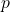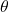# Variational Inference (part 1)

Andy Miller

I will dedicate the next few posts to variational inference methods as a way to organize my own understanding – this first one will be pretty basic.

The goal of variational inference is to approximate an intractable probability distribution,, with a tractable one,, in a way that makes them as ‘close’ as possible. Let’s unpack that statement a bit.

1. Intractable: a motivating example is the posterior distribution of a Bayesian model, i.e. given some observationsand some modelparameterized by, we often want to evaluate the distribution over parametersFor a lot of interesting models this distribution is intractable to deal with because of the integral in the denominator. We can evaluate the posterior up to a constant, but we can’t compute the normalization constant. Applying variational inference to posterior distributions is sometimes called variational Bayes.

2. A tractable posterior distribution is one for which we can evaluate the integral (and therefore take expectations with it). One way to achieve this is by making eachindependent:for some marginal distributionsparameterized by. This is called the ‘mean field’ approximation.

3. We can make two distributions ‘close’ in the Kullback-Leibler sense:Notice that we choseand notbecause of the intractability – we’re assuming you cannot evaluate.

In order to minimize the KL divergence, note the following decompositionwherefalls out of the expectation with respect to. This is convenient because(the log evidence) is going to be constant with respect to the variational distributionand the model parameters.

And because KL divergence is strictly nonnegative, the second termis a lower bound for, also known as the evidence lower bound (ELBO). In order to minimize the first term,, it suffices to maximize the second.

One way to optimize over the choice ofis to consider the ELBO with respect some, separating it from the expectation with respect to all other variables,(note thatis the integral over allwith):whereThis motivates a particular updating scheme: iterate over the marginals, maximizing the ELBO at each step with, and repeat.

These statements remain pretty general. We haven’t specified the functional form of, but it will fall out of thefor specific models (a good, simple example is the normal-gamma model from Murphy’s MLAPP or Bishop’s PRML). This form will then define the variational parameters, and the iterative algorithm will provide a way to compute them.

Some fun properties of the mean field approximation:

• The optimization function is not guaranteed to be convex (wainwright and jordan)
• The optimization procedure is pretty much out of the box for exponential family models (maybe a future blog post will be dedicated to exploring this)
• This variational approximation underestimates uncertainty (a consequence that pops out as a result of the KL divergence ordering,as opposed to).

This begs the question, can you do better with richerdistributions? Yes! In future posts, I will take a look at some more complicated variational distributions and their performance.

references:

1. Kevin Murphy. Machine learning: A probabilistic perspective
2. Wainwright and Jordan. Graphical Models, Exponential Families, and Variational Inference